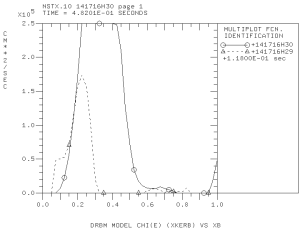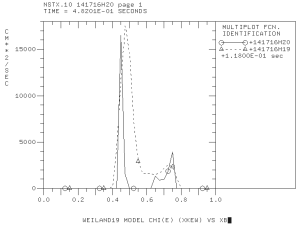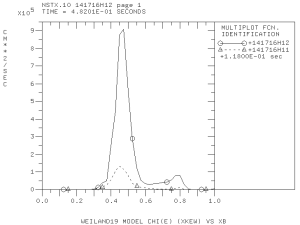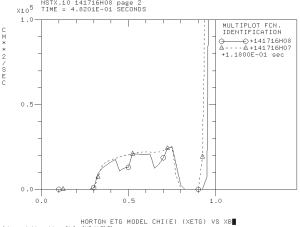## Some observations, conclusions, and plans

I have several observations:

1. The default settings for$k_yrho$ are suppose to correspond the most unstable modes. However, these setting were found in scans for conventional tomakak parameters (primary for H-mode parameters at DIII-D). These default settings do not necessarily correspond to the most unstable modes at NSTX.
2. The modes with different$k_yrho$ might be more unstable in different plasma regions. The DRIBM model with default parameter$k_yrho=0.2$ does not show any anomalous transport in the plasma core for$rho< 0.3$. However, we found that there might be anomalous transport driven by smaller scale instabilities that have$k_yrho=0.6$.

In order to address these observations, several improvements in the MMM8.1 model can be considered. These improvements include:

1. Loops over spectrum of$k_yrho$ needs to be implemented for DRIBM and Weiland models. Individual values of$k_yrho$ that correspond to maximum growth rates need to be selected for every flux surface for every numerical iteration. Depending on the$k_yrho$ ranges and granularity, these change will make the Multi-Mode model order of magnitude slower, but it will increase the robustness of the results.
2. The ExB flow shear effect should depend on$k_yrho$ value. There is no theory-based model at this time that can be implemented in MMM8.1. We can develop such a model by running gyro-kinetic simulations (for example, using the GYRO code). We can perform several scans with respect to$k_yrho$ values. Using these scan, we can develop a parametrized dependencies of the ExB flow shear factor with respect to$k_yrho$.

These developments introduce significant changes to the model and are not currently funded. They might be included as new tasks to the NSTX proposal.

Next steps:

1. Compile the scan with respect to$k_yrho$ and ExB flow shear factor
2. Run the PTRANSP simulations with adjusted$k_yrho$ and ExB flows shear factors from t=0.364 sec to t=0.482 sec and to compare the predicted temperature profiles with the experimental observations.
3. Compare the diffusivities from the Multi-Mode model with the diffusivities from other models (TGLF).
4. Verify the ETG model results with other models (GLF23, TGLF and possibly GYRO).

## Several new cases that complete the scan with respect to (k_y rho)

I have completed two additional cases with$k_yrho=0.6$ (case 141716H29/30) and with$k_yrho=6$ (case 141716H27/28) for DRIBM. Probably, the later case stretches the applicability of DRIBM model somewhat. I used the ExB flow shear factor 0.3 for the case 141716H29/30 and 0.1 for the case 141716H27/28. There very a small electron thermal transport predicted in the case 141716H27/28. The results for the case 141716H29/30 are given below:There is large a level of anomalous transport for modes with$k_yrho=0.6$. The level of anomalous transport exceeds predictions from the model that use the default settings$k_yrho=0.2$ and$k_yrho=0.316$ for Weiland.

## Summary of recent TRANSP runs

Here is the summary of recent PTRANSP runs

141716H09/10 ExB flow shear is enabled for W19 and disabled for DRIBM
141716H11/12 ExB flow shear is disabled for W19 and DRIBM
141716H13/14 MMM95 is used instead of MMM7.1 relatively small changes in diffusivities
141716H17/18 MMM7.1 with ExB flow shear effect for DRIBM ITG/TEM diffusivities goes almost to zero with current levels ExB flow shear effects
141716H19/20 ExB flow shear factor is reduced from 1 to 0.5 for W19 and DRIBM see comment below
141716H21/22 flow shear factor is increased from 0.5 to 0.75
141716H23/24$k_yrho$ in DRIBM is increased from 0.2 to 2 see comments below
141716H25/26 flow shear factor is reduced from 0.75 to 0.5 for W19 and to 0.1 for DRIBM see comments below

There are various definitions of$omega_{rm Etimes B}$ including the Hahm-Burrell and Waltz-Miller definitions. The flow shear rates computed using different definitions are often varied by a factor of 2 or more. The effect of ExB flow shear on particular modes in tokamak even is less certain and requires detailed studies. I plan to vary the ExB flow shear factor from 0.1 to 1 in my simulations depending on the scales of particular modes. In particular, I reduce the ExB flow shear factor to 0.1, when I change$k_yrho$ from 0.2 to 2, but I keet the ExB flow shear factor 0.5 for W19 that has$k_yrho=0.316$ in the PTRANSP simulations 141716H25 and 141716H26.

Some results from my PTRANSP simulations are below.
Case 141716H19/20The effect of ExB flow shear is clearly visible if these results are compared with the results from the case 141761H11/12 that does not take into account the ExB flow shear effect for W19:Without the ExB flow shear, the ITG/TEM modes become more unstable at later time. Account for the ExB flow shear makes these modes more stable at the later time.
Case 141716H23/24Reduction of the characteristic scales of modes with$k_yrho$ increased from 0.2 to 2.0 resulted in a significant level of anomalous transport in the plasma core that has not predicted with the W19 or DRIBM model with the default$k_yrho$ parameters. The ExB flow shear factor should be probably less in these simulations because such short-scale modes should not be affected by the ExB flow shear at the same level as modes with$k_yrho=0.2$. I reduced the ExB flow shear factor for these modes from 0.5 to 0.1 in the next case 141716H25/26.
Case 141716H25/26## Testing of the Weiland, ETG and DRIBM models for the NSTX discharge 141716

Several short PTRANSP simulations are performed to test the effect of the ExB flow shear on Weiland (ITG/TEM) and DRIBM diffusivities. The PTRANSP code has been run for a very short time to ensure that the plasma profiles do not change significantly from the experimental profiles. This choice of the PTRANSP parameters will allow direct comparison of the effective diffusivities, computed using the predictive transport models, with the diffusivities obtained in an interpretive TRANSP runs. [The TRANSP code does not support running predictive transport models when it runs in the interpretive mode.]

I used the following PTRANSP parameters in my simulation:

TINIT=0.482 !**** START TIME
FTIME=0.48201  !**** STOP TIME
DTMINT=4.e-6
DTMAXB=1.e-5
DTMAXT=1.e-5
DTINIT=1.e-5
TBONMIN=0.80 !     !**** MINIMUM BEAM TURN ON TIME

For the first set of simulations the start time was TINIT=0.364 and for the second set the start time was TINIT=0.482. For the both sets, the simulations run for 1e-5 sec. There were no sources in these simulations enabled. The objective was to estimate the predictive diffusivities for the experimental profiles and to validate some trends in the diffusivity profiles between the two time slices.

I found that the that the ETG diffusivities change very little between the two time slices.The result is somewhat unexpected because the plasma profiles are rather different:

While the electron density gradients change very little, there is a large increase of electron temperature gradient in the region from$rho=0.3$ to$rho=0.5$. We assume that there is almost no flow shear effect in the ETG diffusivities and the ETG model does not include the ExB flow shear effect.

## TRANSP modeling of NSTX discharge 141716

Two sets of simulations are proposed for the NSTX discharge 141716. The first set corresponds to the plasma parameters at t=0.364 sec. The second set corresponds to the plasma parameters at t=0.482 sec. The values of ExB flow shear was significantly increased between these two time slices in the regions from$rho=0.35$ to$rho=0.70$ and around$rho=0.80$.

The objective is to test the applicability of the MMM 8.1 anomalous transport model under different values of the ExB flow shear. It is expected that the growth rates and effective diffusivities are much smaller at the later time, when the ExB flow shear rates are significantly larger. The NSTX experiment suggests that this statement is true for short scale instabilities as well.